# 向图中添加文本

### 文本位置

x = linspace(0,10,50); y = sin(x); plot(x,y) txt = '\leftarrow sin(\pi) = 0'; text(pi,sin(pi),txt)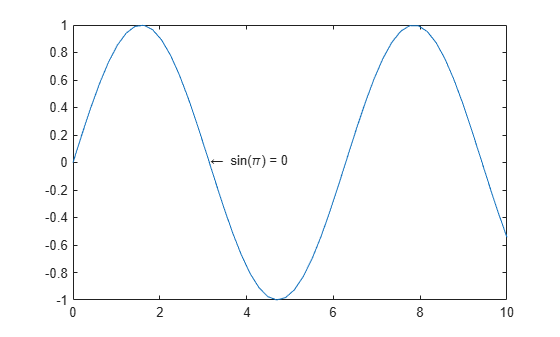### 文本对齐方式

x = linspace(0,10,50); y = sin(x); plot(x,y) txt = 'sin(\pi) = 0 \rightarrow'; text(pi,sin(pi),txt,'HorizontalAlignment','right')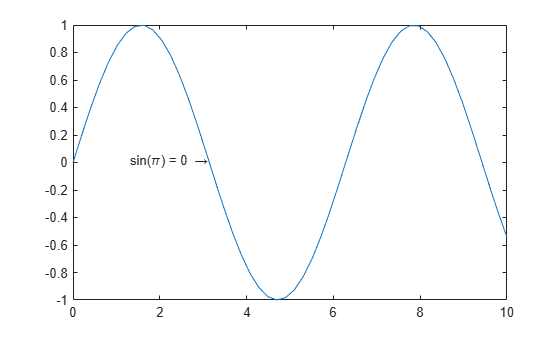### 字体大小

x = linspace(0,10,50); y = sin(x); plot(x,y) txt = '\leftarrow sin(\pi) = 0'; text(pi,sin(pi),txt,'FontSize',14)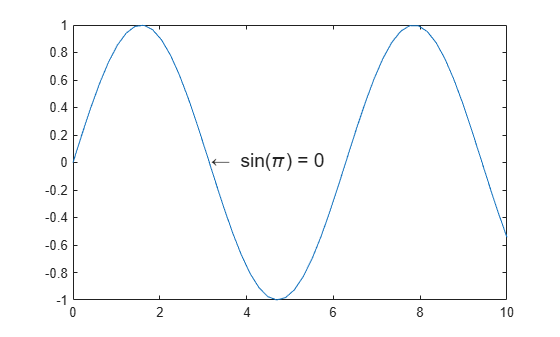### 设置文本属性

text 函数用于创建 Text 对象。Text 对象具有可用来自定义文本外观的属性，例如 HorizontalAlignmentFontSize

• text 命令中使用名称-值对组，例如 'FontSize',14

• 使用 Text 对象。您可以将 Text 对象作为 text 函数的输出参数返回，并将其赋给某个变量，例如 t。然后，使用圆点表示法设置属性，例如 t.FontSize = 14

x = linspace(0,10,50); y = sin(x); plot(x,y) txt = '\leftarrow sin(\pi) = 0'; t = text(pi,sin(pi),txt)
t = Text (\leftarrow sin(\pi) = 0) with properties: String: '\leftarrow sin(\pi) = 0' FontSize: 10 FontWeight: 'normal' FontName: 'Helvetica' Color: [0 0 0] HorizontalAlignment: 'left' Position: [3.1416 1.2246e-16 0] Units: 'data' Show all properties 
t.FontSize = 14;### 多行文本

x = linspace(0,10,50); y = sin(x); plot(x,y) txt = {'Plotted Data:','y = sin(x)'}; text(4,0.5,txt)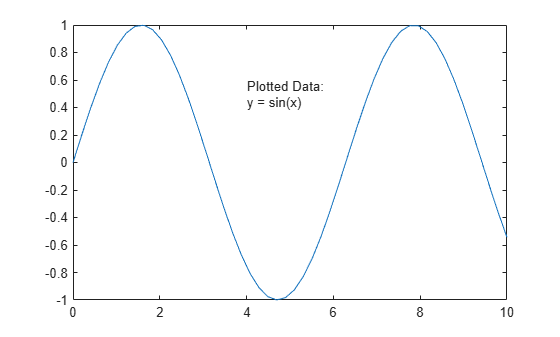### 带有变量值的文本

x = linspace(0,10,50); y = sin(x); plot(x,y) avg = mean(y); txt = ['Average height: ' num2str(avg) ' units']; text(4,0.5,txt)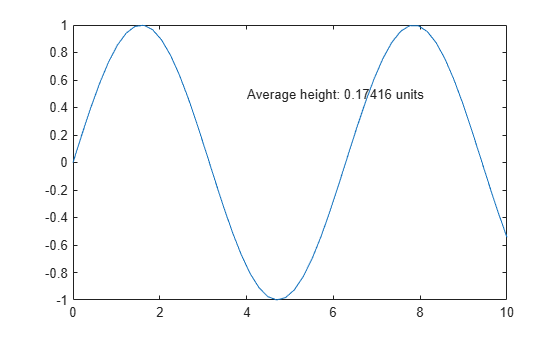### 坐标区外部的文本

x = linspace(0,10,50); y = sin(x); plot(x,y) annotation('textbox',[.9 .5 .1 .2], ... 'String','Text outside the axes','EdgeColor','none')